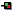## Some Other Points

### Static Imports

Static imports allow us to import constants or static functions that are defined in other classes. The main benefit is that it can make our code slightly more readable. For example if we have a regular segment of code, where we use a static constant and a static method from the`Math` class, like:

```import java.lang.Math; //implicit
public class Test {
public static void main(String args[])
{
double x = Math.PI * Math.sqrt(25.0d);
}
}
```

We can use static imports to rewrite this code as:

```import static java.lang.Math.PI;
import static java.lang.Math.sqrt;

public class Test {
public static void main(String args[])
{
double x = PI * sqrt(25.0d);
}
}
```

So, in the second example there was no need for the `Math` prefix before `PI` or `sqrt()`.

### Autoboxing

Autoboxing was added to Java (JDK 1.5)to provide the ability to convert easily between a variable of a primitive type (e.g. `int`) and its wrapper (e.g.`Integer`).You can think of autoboxing as the automatic conversion of an `int` to an object of its wrapper in an automatic way. For example:

```public class TestAutoboxing {

//f() is only static because being called by main()
static void f(int x){
System.out.println("The output is: " + x);
}

public static void main(String args[]){
Integer y = 10;
Integer z = y + 20; //Integer y automatically converted to an int
f(z);  //Integer z automatically converted to an int
}
}```